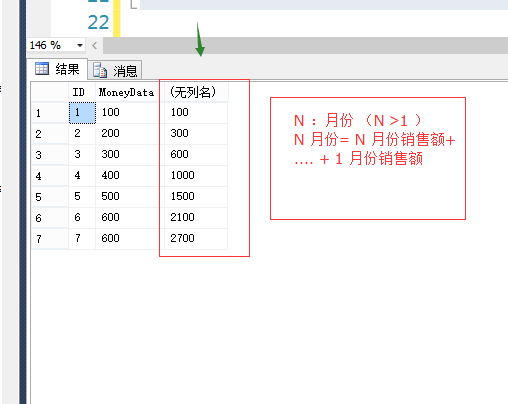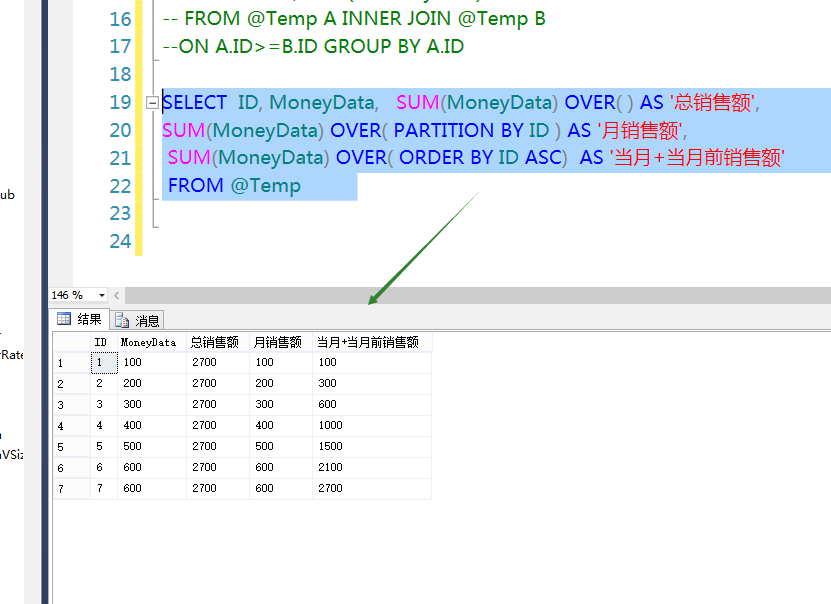# SQL SERVER：开窗函数 SUM() OVER() 数据统计中一例使用

https://www.cnblogs.com/Lumia1020/p/5439982.htmlSQL 测试表脚本

 1 2 3 4 5 6 7 8 9 10 11 12 13 14 `DECLARE` `@``Temp` `Table` `(` `  ``ID ``INT``, ``--- 月份` `  ``MoneyData ``Float`  `--- 金额` `)` `insert`  `INTO` `@``TEMP` `SELECT` `1,100 ``UNION` `ALL` `SELECT`  `2,200 ``UNION` `ALL` `SELECT`  `3,300 ``UNION` `ALL` `SELECT`  `4,400 ``UNION` `ALL` `SELECT`  `5,500 ``UNION` `ALL` `SELECT`  `6,600 ``UNION` `ALL` `SELECT`  `7,600`

一 自连接

 1 2 3 4 5 `SELECT`  `A.ID, ``SUM``(B.MoneyData)  ``FROM` `@``Temp` `A ``INNER` `JOIN` `@``Temp` `B` `ON` `A.ID>=B.ID ``GROUP` `BY` `A.ID` `------ 重点在于 ON 条件，通过自连接 A.ID >= B.ID ，可获取所需数据，再通过` `GROUP` `BY` `、``SUM` `即可统计求和`

 1 2 3 4 5 6 `SELECT`  `ID, MoneyData,   ``SUM``(MoneyData) OVER( ) ``AS` `'总销售额'``,` `SUM``(MoneyData) OVER( PARTITION ``BY` `ID ) ``AS` `'月销售额'``,` ` ``SUM``(MoneyData) OVER( ``ORDER` `BY` `ID ``ASC``)  ``AS` `'当月+当月前销售额'` ` ``FROM` `@``Temp` `---- 此处，使用开窗函数使用更为简单，不过，其中 SUM() OVER ( ORDER BY ID ASC ) 即可实现以上功能`